# The HPGENSELECT Procedure

#### Algorithm Descriptions

The following subsections provide details about each optimization technique and follow the same order as Table 7.10.

##### Trust Region Optimization (TRUREG)

The trust region method uses the gradient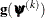and the Hessian matrix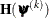; thus, it requires that the objective function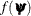have continuous first- and second-order derivatives inside the feasible region.

The trust region method iteratively optimizes a quadratic approximation to the nonlinear objective function within a hyperelliptic trust region with radius
that constrains the step size that corresponds to the quality of the quadratic approximation. The trust region method is implemented based on Dennis, Gay, and Welsch (1981); Gay (1983); Moré and Sorensen (1983).

The trust region method performs well for small- to medium-sized problems, and it does not need many function, gradient, and Hessian calls. However, if the computation of the Hessian matrix is computationally expensive, one of the dual quasi-Newton or conjugate gradient algorithms might be more efficient.

##### Newton-Raphson Optimization with Line Search (NEWRAP)

The NEWRAP technique uses the gradientand the Hessian matrix; thus, it requires that the objective function have continuous first- and second-order derivatives inside the feasible region.

If second-order derivatives are computed efficiently and precisely, the NEWRAP method can perform well for medium-sized to large problems, and it does not need many function, gradient, and Hessian calls.

This algorithm uses a pure Newton step when the Hessian is positive-definite and when the Newton step reduces the value of the objective function successfully. Otherwise, a combination of ridging and line search is performed to compute successful steps. If the Hessian is not positive-definite, a multiple of the identity matrix is added to the Hessian matrix to make it positive-definite (Eskow and Schnabel, 1991).

In each iteration, a line search is performed along the search direction to find an approximate optimum of the objective function. The line-search method uses quadratic interpolation and cubic extrapolation.

##### Newton-Raphson Ridge Optimization (NRRIDG)

The NRRIDG technique uses the gradientand the Hessian matrix; thus, it requires that the objective function have continuous first- and second-order derivatives inside the feasible region.

This algorithm uses a pure Newton step when the Hessian is positive-definite and when the Newton step reduces the value of the objective function successfully. If at least one of these two conditions is not satisfied, a multiple of the identity matrix is added to the Hessian matrix.

Because the NRRIDG technique uses an orthogonal decomposition of the approximate Hessian, each iteration of NRRIDG can be slower than an iteration of the NEWRAP technique, which works with a Cholesky decomposition. However, NRRIDG usually requires fewer iterations than NEWRAP.

The NRRIDG method performs well for small- to medium-sized problems, and it does not require many function, gradient, and Hessian calls. However, if the computation of the Hessian matrix is computationally expensive, one of the dual quasi-Newton or conjugate gradient algorithms might be more efficient.

##### Quasi-Newton Optimization (QUANEW)

The dual quasi-Newton method uses the gradient, and it does not need to compute second-order derivatives because they are approximated. It works well for medium-sized to moderately large optimization problems, where the objective function and the gradient can be computed much faster than the Hessian. However, in general the QUANEW technique requires more iterations than the TRUREG, NEWRAP, and NRRIDG techniques, which compute second-order derivatives. The QUANEW technique provides an appropriate balance between the speed and stability that are required for most generalized linear model applications.

The QUANEW technique that is implemented by the HPGENSELECT procedure is the dual quasi-Newton algorithm, which updates the Cholesky factor of an approximate Hessian.

In each iteration, a line search is performed along the search direction to find an approximate optimum. The line-search method uses quadratic interpolation and cubic extrapolation to obtain a step size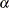that satisfies the Goldstein conditions (Fletcher, 1987). One of the Goldstein conditions can be violated if the feasible region defines an upper limit of the step size. Violating the left-side Goldstein condition can affect the positive-definiteness of the quasi-Newton update. In that case, either the update is skipped or the iterations are restarted by using an identity matrix, resulting in the steepest descent or ascent search direction.

##### Double-Dogleg Optimization (DBLDOG)

The double-dogleg optimization method combines the ideas of the quasi-Newton and trust region methods. In each iteration, the double-dogleg algorithm computes the step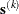as the linear combination of the steepest descent or ascent search direction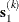and a quasi-Newton search direction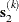: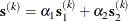The step is requested to remain within a prespecified trust region radius (Fletcher, 1987, p. 107). Thus, the DBLDOG subroutine uses the dual quasi-Newton update but does not perform a line search.

The double-dogleg optimization technique works well for medium-sized to moderately large optimization problems, where the objective function and the gradient can be computed much faster than the Hessian. The implementation is based on Dennis and Mei (1979); Gay (1983), but it is extended for dealing with boundary and linear constraints. The DBLDOG technique generally requires more iterations than the TRUREG, NEWRAP, and NRRIDG techniques, which require second-order derivatives; however, each of the DBLDOG iterations is computationally cheap. Furthermore, the DBLDOG technique requires only gradient calls for the update of the Cholesky factor of an approximate Hessian.

##### Conjugate Gradient Optimization (CONGRA)

Second-order derivatives are not required by the CONGRA algorithm and are not even approximated. The CONGRA algorithm can be expensive in function and gradient calls, but it requires only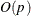memory for unconstrained optimization. In general, the algorithm must perform many iterations to obtain a precise solution, but each of the CONGRA iterations is computationally cheap.

The CONGRA algorithm should be used for optimization problems that have large p. For the unconstrained or boundary-constrained case, the CONGRA algorithm requires onlybytes of working memory, whereas all other optimization methods require order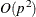bytes of working memory. During p successive iterations, uninterrupted by restarts or changes in the working set, the CONGRA algorithm computes a cycle of p conjugate search directions. In each iteration, a line search is performed along the search direction to find an approximate optimum of the objective function. The line-search method uses quadratic interpolation and cubic extrapolation to obtain a step sizethat satisfies the Goldstein conditions. One of the Goldstein conditions can be violated if the feasible region defines an upper limit for the step size.

##### Nelder-Mead Simplex Optimization (NMSIMP)

The Nelder-Mead simplex method does not use any derivatives and does not assume that the objective function has continuous derivatives. The objective function itself needs to be continuous. This technique is quite expensive in the number of function calls, and it might be unable to generate precise results for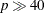.

The original Nelder-Mead simplex algorithm is implemented and extended to boundary constraints. This algorithm does not compute the objective for infeasible points, but it changes the shape of the simplex adapting to the nonlinearities of the objective function. This change contributes to an increased speed of convergence and uses a special termination criterion.